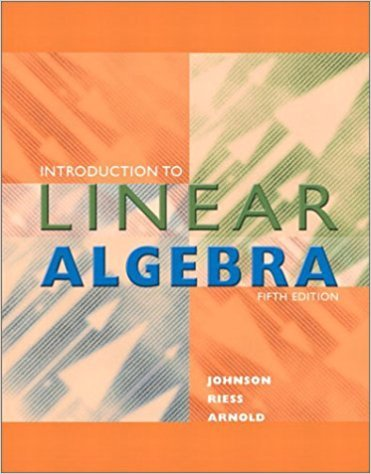×
×

# Solutions for Chapter A.2: Introduction to Linear Algebra 5th Edition## Full solutions for Introduction to Linear Algebra | 5th Edition

ISBN: 9780201658590Solutions for Chapter A.2

Solutions for Chapter A.2
4 5 0 292 Reviews
28
1
##### ISBN: 9780201658590

This textbook survival guide was created for the textbook: Introduction to Linear Algebra , edition: 5. Introduction to Linear Algebra was written by and is associated to the ISBN: 9780201658590. This expansive textbook survival guide covers the following chapters and their solutions. Since 1 problems in chapter A.2 have been answered, more than 7464 students have viewed full step-by-step solutions from this chapter. Chapter A.2 includes 1 full step-by-step solutions.

Key Math Terms and definitions covered in this textbook
• Companion matrix.

Put CI, ... ,Cn in row n and put n - 1 ones just above the main diagonal. Then det(A - AI) = ±(CI + c2A + C3A 2 + .•. + cnA n-l - An).

• Covariance matrix:E.

When random variables Xi have mean = average value = 0, their covariances "'£ ij are the averages of XiX j. With means Xi, the matrix :E = mean of (x - x) (x - x) T is positive (semi)definite; :E is diagonal if the Xi are independent.

• Diagonalization

A = S-1 AS. A = eigenvalue matrix and S = eigenvector matrix of A. A must have n independent eigenvectors to make S invertible. All Ak = SA k S-I.

• Dimension of vector space

dim(V) = number of vectors in any basis for V.

• Dot product = Inner product x T y = XI Y 1 + ... + Xn Yn.

Complex dot product is x T Y . Perpendicular vectors have x T y = O. (AB)ij = (row i of A)T(column j of B).

• Elimination matrix = Elementary matrix Eij.

The identity matrix with an extra -eij in the i, j entry (i #- j). Then Eij A subtracts eij times row j of A from row i.

• Fourier matrix F.

Entries Fjk = e21Cijk/n give orthogonal columns FT F = nI. Then y = Fe is the (inverse) Discrete Fourier Transform Y j = L cke21Cijk/n.

• Full column rank r = n.

Independent columns, N(A) = {O}, no free variables.

• Gauss-Jordan method.

Invert A by row operations on [A I] to reach [I A-I].

• Graph G.

Set of n nodes connected pairwise by m edges. A complete graph has all n(n - 1)/2 edges between nodes. A tree has only n - 1 edges and no closed loops.

• Identity matrix I (or In).

Diagonal entries = 1, off-diagonal entries = 0.

• Left nullspace N (AT).

Nullspace of AT = "left nullspace" of A because y T A = OT.

• Nullspace N (A)

= All solutions to Ax = O. Dimension n - r = (# columns) - rank.

• Orthogonal subspaces.

Every v in V is orthogonal to every w in W.

• Orthonormal vectors q 1 , ... , q n·

Dot products are q T q j = 0 if i =1= j and q T q i = 1. The matrix Q with these orthonormal columns has Q T Q = I. If m = n then Q T = Q -1 and q 1 ' ... , q n is an orthonormal basis for Rn : every v = L (v T q j )q j •

• Saddle point of I(x}, ... ,xn ).

A point where the first derivatives of I are zero and the second derivative matrix (a2 II aXi ax j = Hessian matrix) is indefinite.

• Schur complement S, D - C A -} B.

Appears in block elimination on [~ g ].

• Spanning set.

Combinations of VI, ... ,Vm fill the space. The columns of A span C (A)!

• Standard basis for Rn.

Columns of n by n identity matrix (written i ,j ,k in R3).

• Tridiagonal matrix T: tij = 0 if Ii - j I > 1.

T- 1 has rank 1 above and below diagonal.

×